# RCTF Reverse 300 Creack Me

```Name: crackMe_aafb0addeb58dece1fcf631a183c2b20 MD5: AAFB0ADDEB58DECE1FCF631A183C2B20 SHA256: 3091F5DF9D1D4E470B36DD8AFBBFEB7F03A5398B3F8846B892425F4BCD890E20 ```

The file of this challenge is a Windows Portable Executable packed with UPX and it can be unpacked with the UPX utility: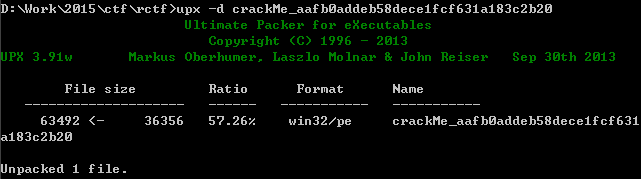The unpacked file introduced some simple anti mechanisms, the first one is located at address 0x0040146E: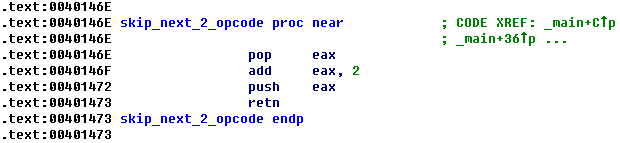The code above will skip the next 2 opcode after this function call. If you did not notice this, you may get confused about the disassembly code.

And the second one is location at address 0x00401468: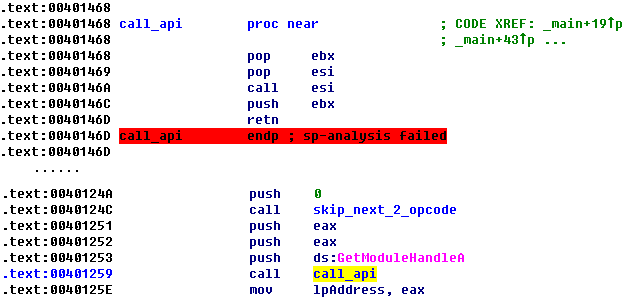This function is used to call the API that passed as an argument. Using this approach to call an API will make some disassembler like IDA unable to recognize the parameters of that API automatically. However, as you can see in above screenshot, it is still very easy to figure out which API will be called through the disassembly code.

It is not difficult to under stand the functionality of this executable, in short, it will decyprt another Portable Executable file from its append data and then execute it in the memory. The decryption algorithm is to XOR each byte with 0x07.

The decrypted PE file also packed with UPX, and it contains the core verification logic of this challenge.

Before we step into the details of the verification logic, there is another thing need to be mentioned: the decrypted file introduced another anti mechanism which will replace the code block that already been executed and no longer needed with random generated data. This is usually seen in malware to hide itself from memory scan.

The decrypted file will read the input data, and then convert it to a hex-like string (sub_401020), the convert rule is:

For each byte of the input data:

(1) If the highest 4 bit is less than or equal to 0x09, add 0x30 to it, otherwise, add it with 0x57. Store the result as a new byte.

(2) If the lowest 4 bit is less than or equal to 0x09, add 0x30 to it, otherwise, add it with 0x57. Store the result as a new byte.

Next, it will encode the hex-like string (sub_401080) with following rule:

(1) Scan the hex-like string for duplicated sub-strings compared with the beginning of the hex-like string.

(2) If no duplicated sub-string is found, each byte will be XORed with 0x18.

(3) If a duplicated sub-string is found, the first byte of the duplicated sub-string will be XORed with 0x19 (0x18+1) and the second byte of the duplicated sub-string will be XORed with 0x1A (0x18+2), and so no. Other bytes in the string will be XORed with 0x18.

For example, for string “ABCDEFABCGHI”, there is a duplicated sub-string “ABC”, so the second “A” in this string will XOR with 0x19, the second “B” in this string will XOR with 0x1A and the second “C” will XOR with 0x1B. And other bytes in this string will XOR with 0x18.

After encode the hex-like string, the encode result will be converted once again to a new hex-like string, and then it will generate a table (see the code from address 0x00401391 to 0x00401435) and change the byte order of the string (sub_4011E0) based on the table generated before.

Finally, it will compare the result from above steps with following string:
``` 22722272222227272222727a2222222222272222272222222222cfdceeeebb9fdbcdbbedfdede7ce9bebe0bb1e2ceab9e2bbbdecf9d8 ```

Now the logic become clear:
`FinalDate = Reorder(ToHex(Encode(ToHex(OriginalData))))`

In order to get the original input, the first thing we should do is to reverse the order of the final data.

So how? Let’s take a look at the function which reorder the data: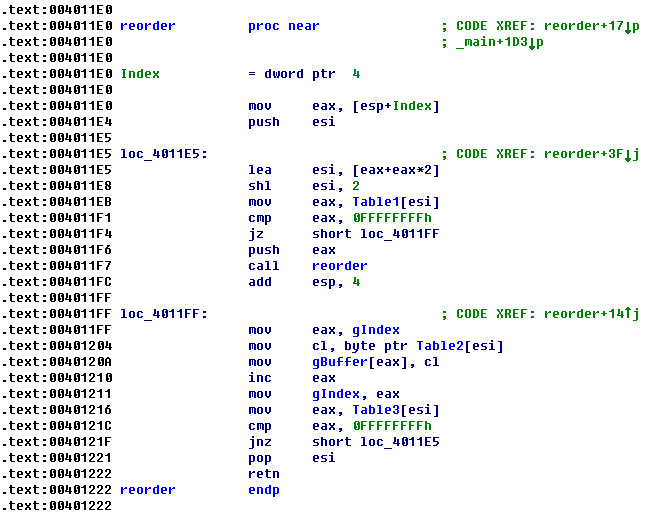By entering some test data into this program we can easily found that if the input data has a same length, the Table1 in above screenshot will keep the same. And the Table2 here contains the second hex-like string described before. The gIndex here is an index value begin from 0.

Since the ToHex() function will double the length of the string and there are two ToHex() function calls, so the length of the original input should be len(FinalDate) / 4 = 27.

Now that we have the length of the input data, we can let the program itself to calculate the Table1 for us, the following immunity debugger script can help with this work:

```import immlib
import getopt
import immutils
from immutils import *
imm = immlib.Debugger()

def main(args):
imm.run()
imm.run()
imm.run()
imm.setBreakpoint(0x00401204)
imm.setBreakpoint(0x00401448)
while True:
imm.run()
regs = imm.getRegs()
esi = regs['ESI']
imm.log(" ESI : %08x" % (esi))
open('d:\work\esi.txt', 'ab').write(hex(esi)+ '\r\n')
if regs['EIP'] == 0x00401448:
break

```

After we get the Table1 we can reverse the final data, and then UnHex and Decode it to get the original input. All the works are can be done by the following Python script:

```def convert(data):

out = ''
datalen = len(data)

for i in range(0, datalen, 2):
tmp = 0
if ord(data[i]) <= 0x39:
tmp = ord(data[i]) - 0x30
else:
tmp = ord(data[i]) - 0x57
tmp = tmp <<4
if ord(data[i+1]) <= 0x39:
tmp |= ord(data[i+1]) - 0x30
else:
tmp |= ord(data[i+1]) - 0x57
out += chr(tmp & 0xff)

return out

enc = '22722272222227272222727a2222222222272222272222222222cfdceeeebb9fdbcdbbedfdede7ce9bebe0bb1e2ceab9e2bbbdecf9d8'
table = [0x4f8, 0x258, 0x108, 0x318, 0x2b8, 0x3d8, 0x378, 0x498, 0x438, 0x60, 0x348, 0x168, 0x4c8, 0x408,
0x138, 0x1c8, 0x198, 0x228, 0x1f8, 0x2e8, 0x288, 0x468, 0x3a8, 0xc, 0x420, 0x180, 0x4e0, 0x90,
0x240, 0x1e0, 0x300, 0x2a0, 0x3c0, 0x360, 0x480, 0x78, 0x270, 0xc0, 0x3f0, 0x330, 0x4b0, 0xa8,
0x450, 0xf0, 0xd8, 0x150, 0x120, 0x210, 0x1b0, 0x390, 0x2d0, 0x0, 0x48c, 0x1ec, 0x9c, 0x2ac,
0x24c, 0x36c, 0x30c, 0x42c, 0x3cc, 0x4ec, 0x24, 0x2dc, 0xfc, 0x45c, 0x39c, 0xcc, 0x4bc, 0x15c,
0x12c, 0x1bc, 0x18c, 0x27c, 0x21c, 0x3fc, 0x33c, 0x18, 0x3b4, 0x114, 0x474, 0x3c, 0x1d4, 0x174,
0x294, 0x234, 0x354, 0x2f4, 0x4d4, 0x414, 0x30, 0x204, 0x54, 0x384, 0x2c4, 0x504, 0x444, 0x48,
0x3e4, 0x84, 0x6c, 0xe4, 0xb4, 0x1a4, 0x144, 0x324, 0x264, 0x4a4]

list1 = list(table)
i = 0
for index in table:
list1[index/12] = enc[i]
i += 1
print ''.join(list1)

out1 = convert(''.join(list1))
print out1

out2 = ''
out3 = ''
out4 = ''
out5 = ''
i = 0
for ch in out1:
out2 += chr(ord(ch) ^ 0x18)

if i % 2 == 0:
out3 += chr(ord(ch) ^ 0x19)
else:
out3 += chr(ord(ch) ^ 0x18)

if i % 2 == 0:
out4 += chr(ord(ch) ^ 0x18)
else:
out4 += chr(ord(ch) ^ 0x19)

if i % 2 == 0:
out5 += chr(ord(ch) ^ 0x19)
else:
out5 += chr(ord(ch) ^ 0x1A)
i += 1
print out2
print out2.encode('hex')

print convert(out2)
print convert(out3)
print convert(out4)
print convert(out5)
```

Please note that since we could not know if there is any duplicated sub-string after the first ToHex() function, so we may need to do some “brute-force” here: we can assume there are one byte long duplicated sub-strings and two bytes long duplicated sub-strings, and we can calculate the Decode result for the two situations respectively.

From the output of above script we can find the correct combination of the flag: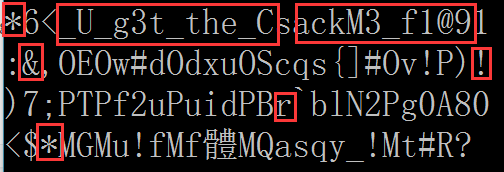Flag: RCTF{*&*_U_g3t_the_CrackM3_f1@9!}

This entry was posted in CTF and tagged , , . Bookmark the permalink.